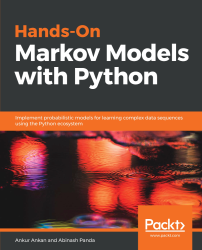•#### Hands-On Markov Models with Python#### Overview of this book

Hidden Markov Model (HMM) is a statistical model based on the Markov chain concept. Hands-On Markov Models with Python helps you get to grips with HMMs and different inference algorithms by working on real-world problems. The hands-on examples explored in the book help you simplify the process flow in machine learning by using Markov model concepts, thereby making it accessible to everyone. Once you’ve covered the basic concepts of Markov chains, you’ll get insights into Markov processes, models, and types with the help of practical examples. After grasping these fundamentals, you’ll move on to learning about the different algorithms used in inferences and applying them in state and parameter inference. In addition to this, you’ll explore the Bayesian approach of inference and learn how to apply it in HMMs. In further chapters, you’ll discover how to use HMMs in time series analysis and natural language processing (NLP) using Python. You’ll also learn to apply HMM to image processing using 2D-HMM to segment images. Finally, you’ll understand how to apply HMM for reinforcement learning (RL) with the help of Q-Learning, and use this technique for single-stock and multi-stock algorithmic trading. By the end of this book, you will have grasped how to build your own Markov and hidden Markov models on complex datasets in order to apply them to projects.
PrefaceFree Chapter
Introduction to the Markov ProcessHidden Markov ModelsState Inference - Predicting the StatesParameter Learning Using Maximum LikelihoodParameter Inference Using the Bayesian ApproachTime Series PredictingNatural Language Processing2D HMM for Image ProcessingMarkov Decision ProcessOther Books You May Enjoy# Markov processes

A stochastic process is called a Markov process if the state of the random variable at the next instance of time depends only on the outcome of the random variable at the current time. In simplistic mathematical terms, for a stochastic process, S = {R1, R2, . . ., Rn} = {R}t=1, . . ., n, to be a Markov process, it must satisfy the following condition:According to the previous condition, the probability distribution for any variable at any given instance in a Markov process is a conditional distribution, which is conditioned only on the random variable at the last time instance. This property of a system, such that the future states of the system depend only on the current state of the system, is also known as the Markov property. Systems satisfying the Markov property are also known as memoryless systems since they don't need to remember the previous states to compute the distribution of the next state, or, in other words, the next state depends only on the current state of the system.

A very common example used to explain the Markov process is a drunk man walking along a street. We consider that, since the man is drunk, he can either take a step backward, a step forward, or stay in his current position, which is given by some distribution of these, let's say [0.4, 0.4, 0.2]. Now, given the position of the man at any given instance in time, his position at the next instance depends only on his current position and the parameters of the system (his step size and the probability distribution of possible actions). Therefore, this is an example of a Markov process.

In the previous example, let's assume that the drunk man takes an action (steps forward/backward or stays in his position) at fixed intervals of time and his step size is always the same. With these considerations, the Markov process in our example has a discrete state space. Also, since the man takes steps after fixed intervals of time, we can think of it as a discrete time. But Markov processes don't need to have discrete state space or discrete time intervals. Considering discrete and continuous time as well as discrete and continuous state space, we can categorize Markov processes into four main categories:

• Discrete time and discrete state space
• Discrete time and continuous state space
• Continuous time and discrete state space
• Continuous time and continuous state space

We will discuss each of these categories of Markov process in more detail in the following sections.